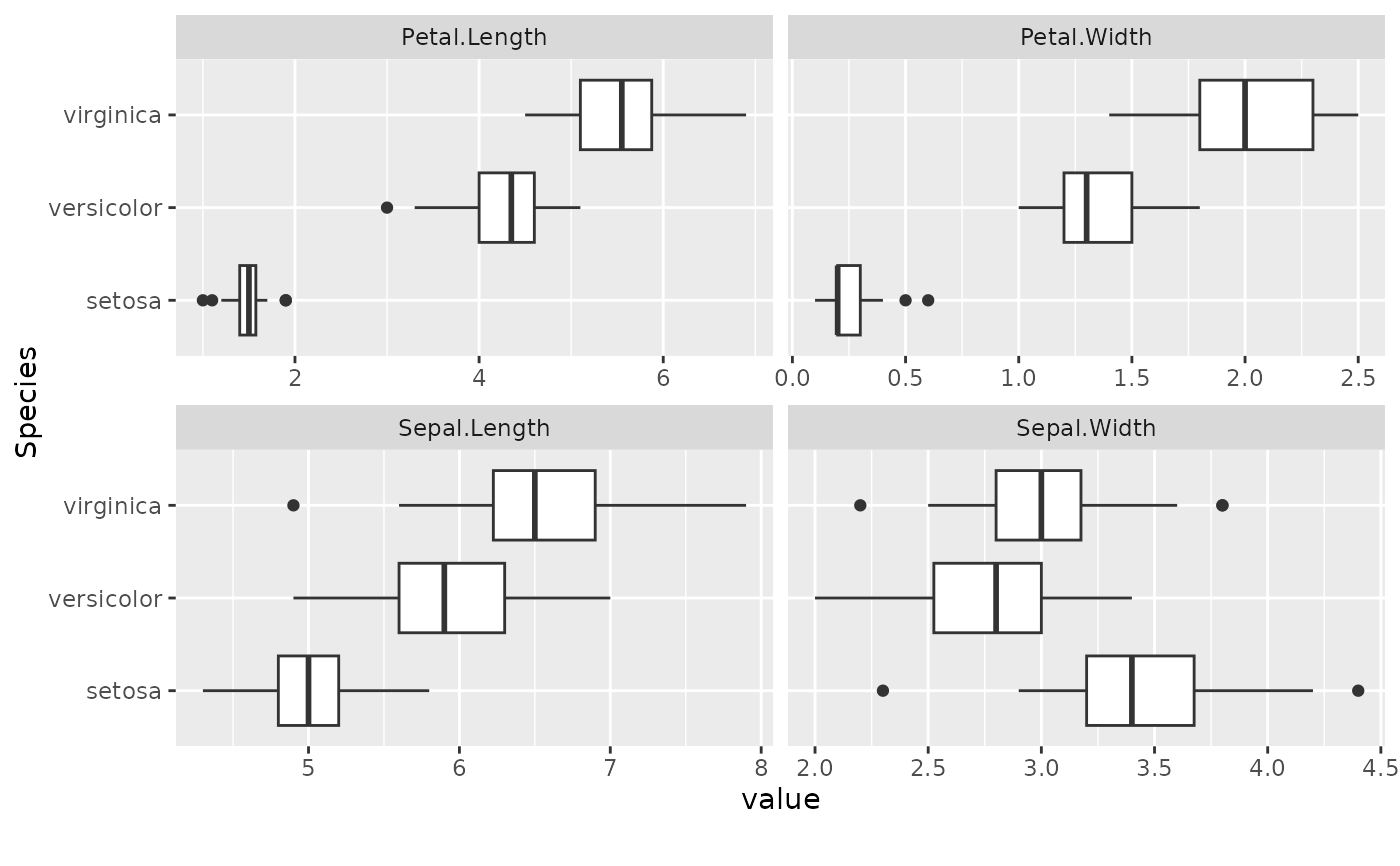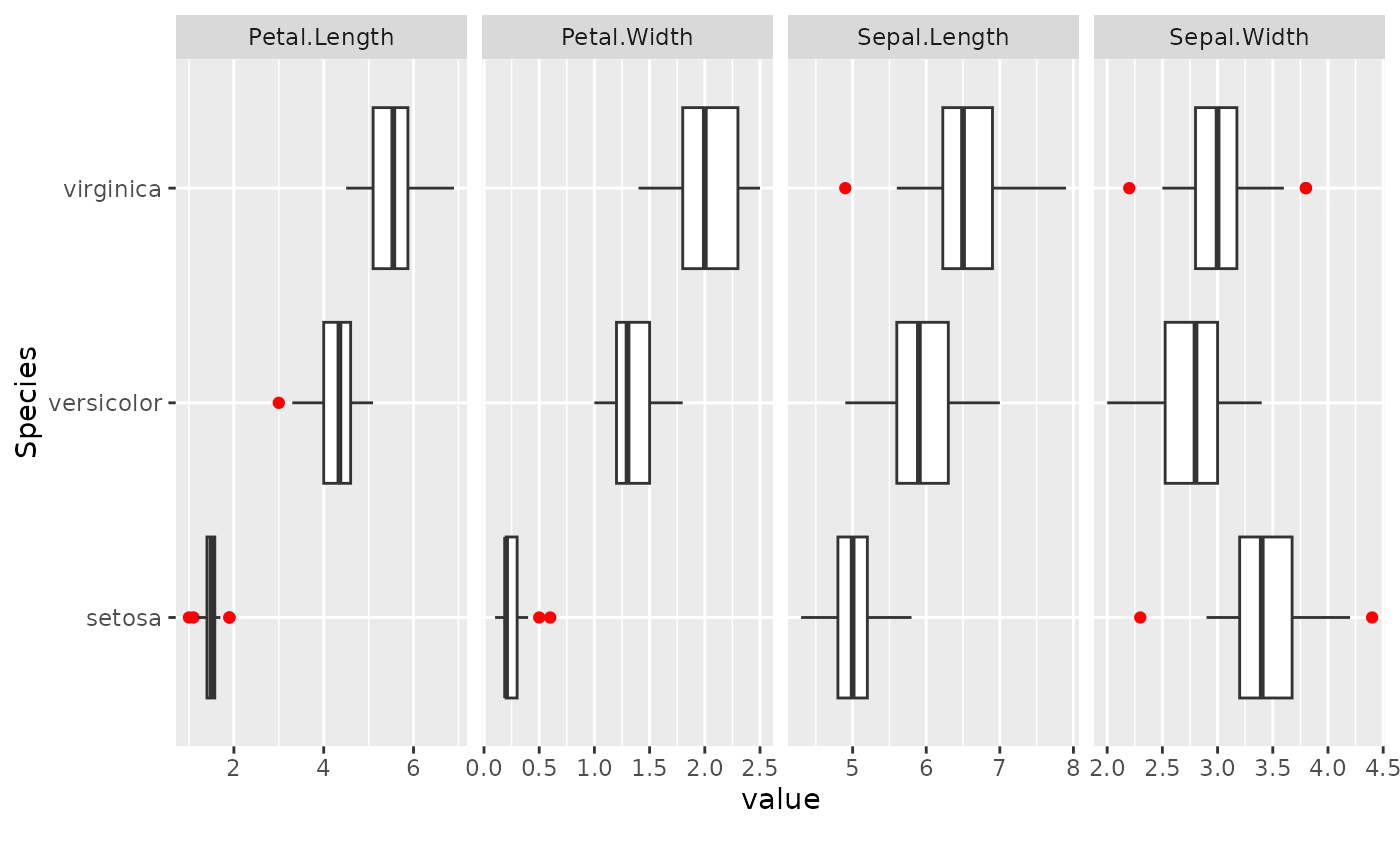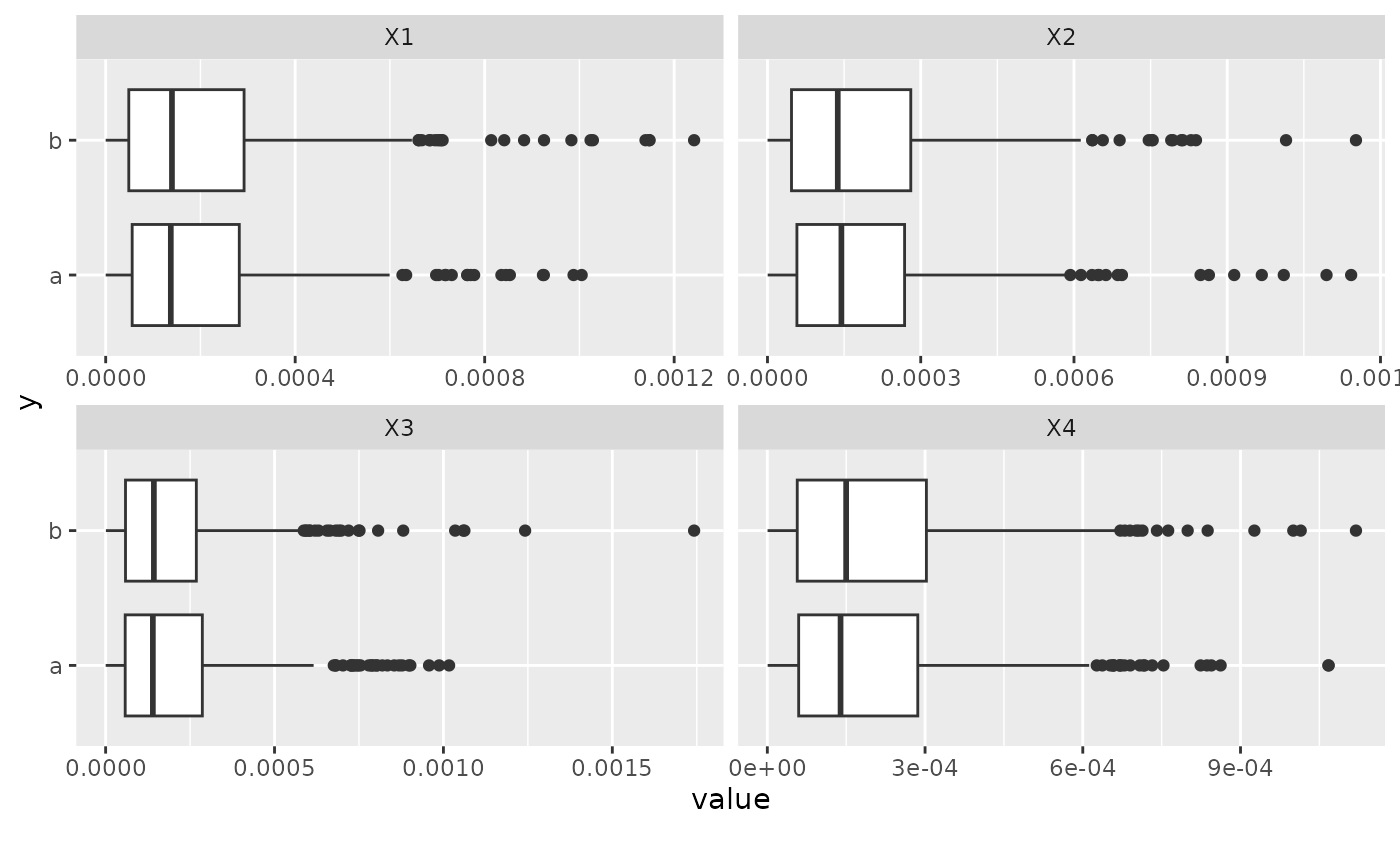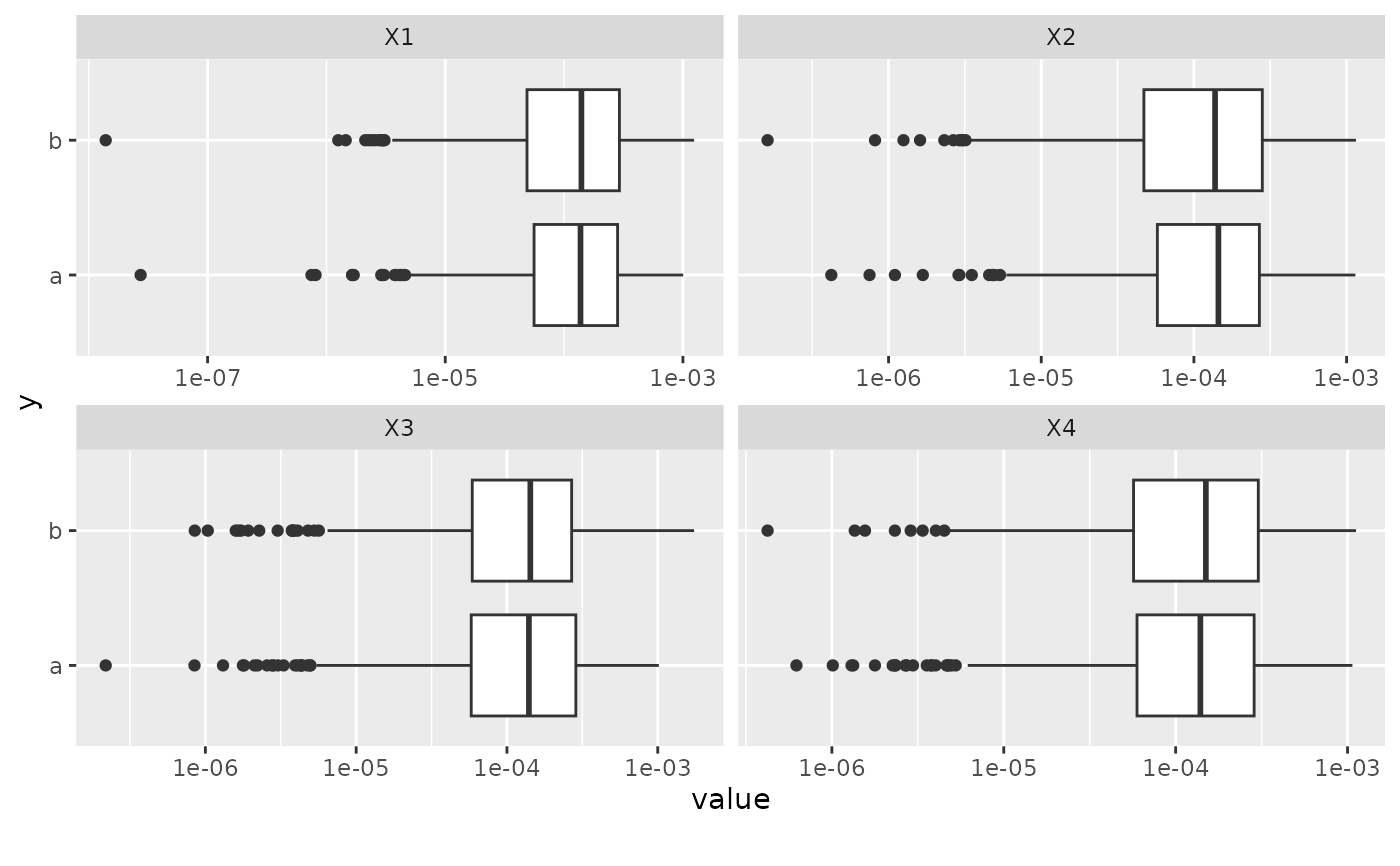This function creates boxplot for each continuous feature based on a selected feature.

plot_boxplot(
data,
by,
binary_as_factor = TRUE,
geom_boxplot_args = list(),
scale_y = "continuous",
title = NULL,
ggtheme = theme_gray(),
theme_config = list(),
nrow = 3L,
ncol = 4L,
parallel = FALSE
)

## Arguments

data input data feature name to be broken down by. If selecting a continuous feature, boxplot will be grouped by 5 equal ranges, otherwise, all existing categories for a discrete feature. treat binary as categorical? Default is TRUE. a list of other arguments to geom_boxplot scale of original y axis (before coord_flip). See scale_y_continuous for all options. Default is continuous. plot title complete ggplot2 themes. The default is theme_gray. a list of configurations to be passed to theme. number of rows per page number of columns per page enable parallel? Default is FALSE.

## Value

invisibly return the named list of ggplot objects

plot_boxplot(iris, by = "Species", ncol = 2L)plot_boxplot(iris, by = "Species", geom_boxplot_args = list("outlier.color" = "red"))plot_boxplot(skew, by = "y", scale_y = "log10", ncol = 2L)